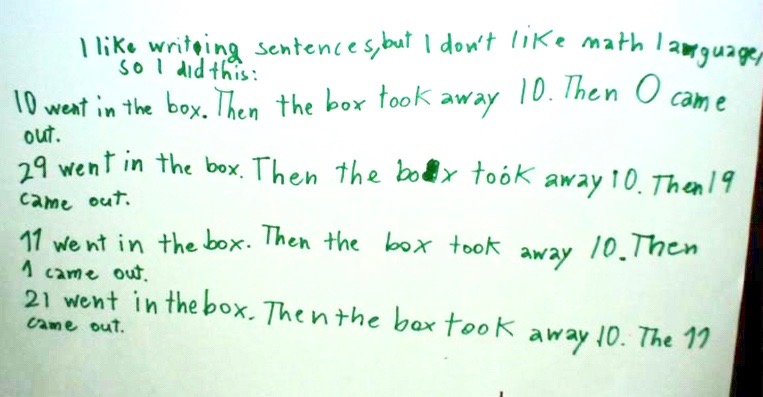#### You may also like### I'm Eight

Find a great variety of ways of asking questions which make 8.### Let's Investigate Triangles

Vincent and Tara are making triangles with the class construction set. They have a pile of strips of different lengths. How many different triangles can they make?### Noah

Noah saw 12 legs walk by into the Ark. How many creatures did he see?

# What Was in the Box?

##### Age 5 to 7Challenge Level

We did not have many solutions sent in showing their thoughts and recording, but here are a few. This first one came from Michael at Cloverdale Catholic School in Canada:

The number that was put into that box was 10, and out came 18. So, therefore, that wonderful big box added 8. (10 + 8 = 18)
Four other boxes were put into that bigger box, and out popped these numbers: 12, 8, 15 and 10. If the box added eight onto ten to make eighteen, then the other numbers must have been added from eight. How do you figure that out? You use the opposite operation, in which in this case it is addition, so we use subtraction. (12-8=4, so 4+8=12) and (8-8=0, so 0+8=8) and etc.

For the other box, one of the boxes that went into the bigger box was 10, and out came the numbers: 0, 19, 1 and 11. If the number 10 was put in and became the number 0, then 10 was subtracted from 10. Thus, 10-10=0, 29-10=19, 11-10=1, and 21-10=11. If 10 became 19, then 9 was added onto ten. Thus, 10+9=19, (-9)+9=0, (-8)+9=1, and 2+9=11. (A more advanced solution would have been multiplying by 1.9, in which case the numbers before the big box would be 0, 10, â‰ˆ0.526 (which means almost 0.526) and â‰ˆ5.789.)

There are two possible ways ten could have turned into one: dividing by 10, or subtracting by 9. In division's case: 10Ã·10=1, 0Ã·10=0, 190Ã·10=19, and 110Ã·10=11. In subtraction's case: 10-9=1, 9-9=0, 28-9=19, and 20-9=11. If 10 was put in that bigger box and became 11, then one was added to ten. Thus, 10+1=11, (-1)+1=0, 18+1=19, and 0+1=1. (An advanced solution is to multiply by 1.1, in which the numbers before the bigger box would have been, â‰ˆ17.27, â‰ˆ0.9 and 10.)

The next one is from Lisa at the Tokyo International School in Japan:

First you have to choose what number/numbers to minus or plus then you choose what box should start with the number. I choose to minus nine in all the boxes. The numbers that come out are 0, 19, 1, 11.
I know that if you take away 9 from ten you get 1. Then you just have to add nine to the rest of the numbers. The number that you get that's the number that you start with. So if you choose to take away the number 9 your solution is 9-9, 28-9, 10-9, 20-9.

Lastly from Louis at the International School, Seychelles:Minette, Freddie, Ursie from Grandtully Primary in Scotland sent in the following:

We kept the number 10 in our heads, if it was a smaller number we knew it was a take away answer and if it was a bigger number we knew it was an add up.

So once we knew if it was a take away or an add up we kept the 10 in our heads and then we counted from 10 to the number it was going to end with and how many that was.

Then that was what happened in the box!
First the box took away 10 to become 0
Then the box added on 9 to become 19
Then the box took away 9 to become 10
Finally the box added on 1 to become 11

Well done those of you who sent in your ideas to solve this challenge, I wonder how many of you went on to have a look at What's in the Box?
----------------